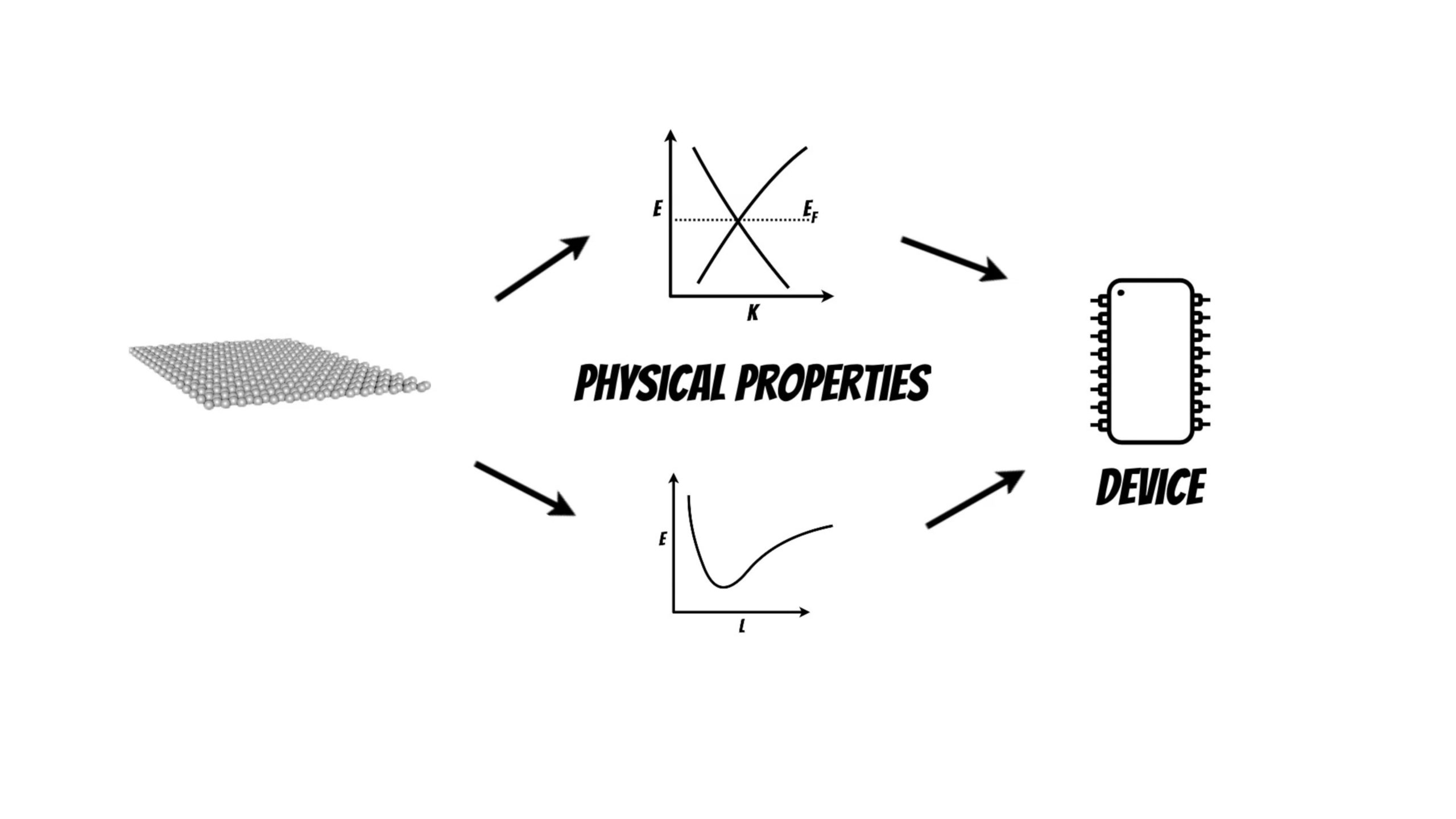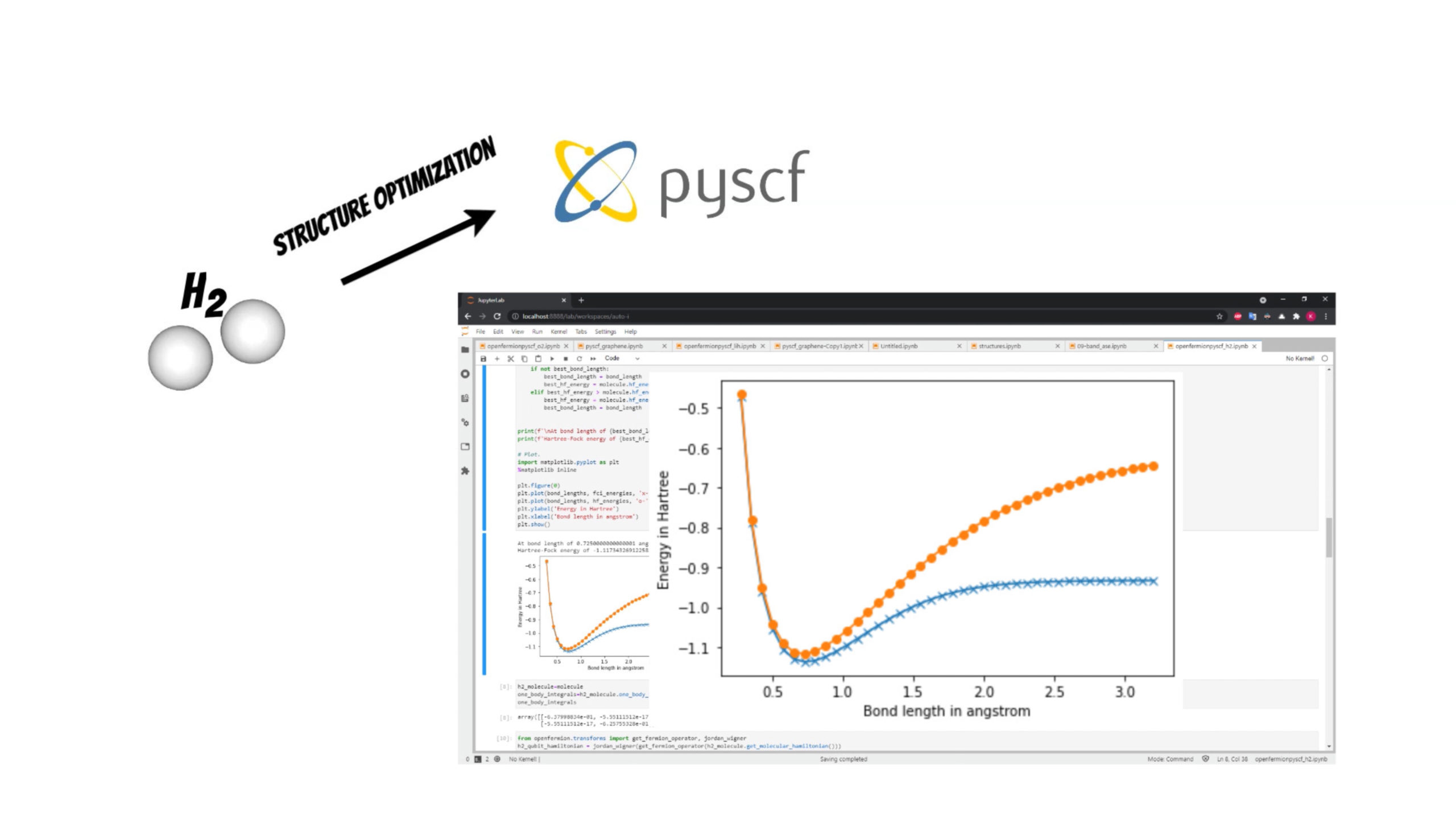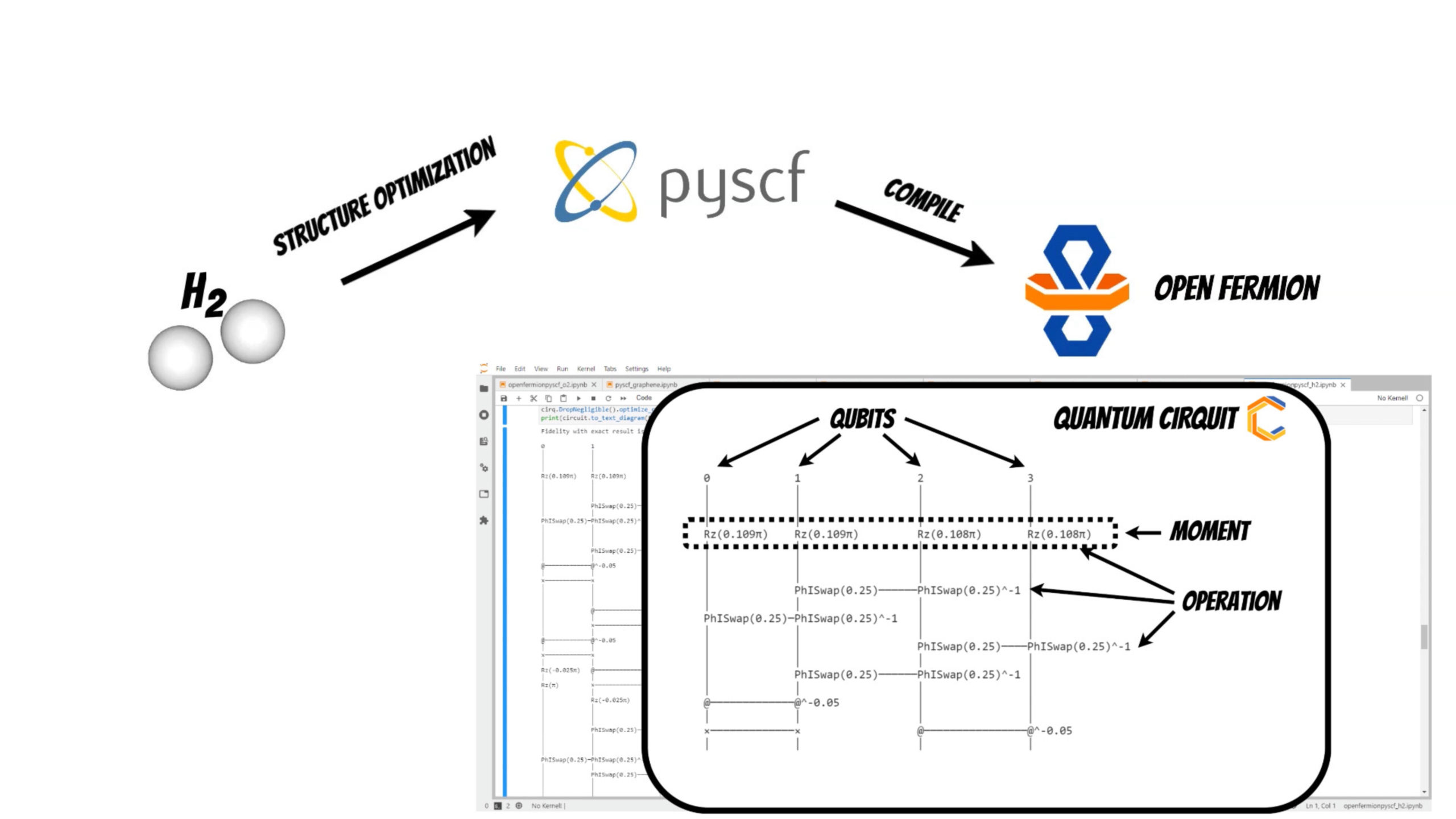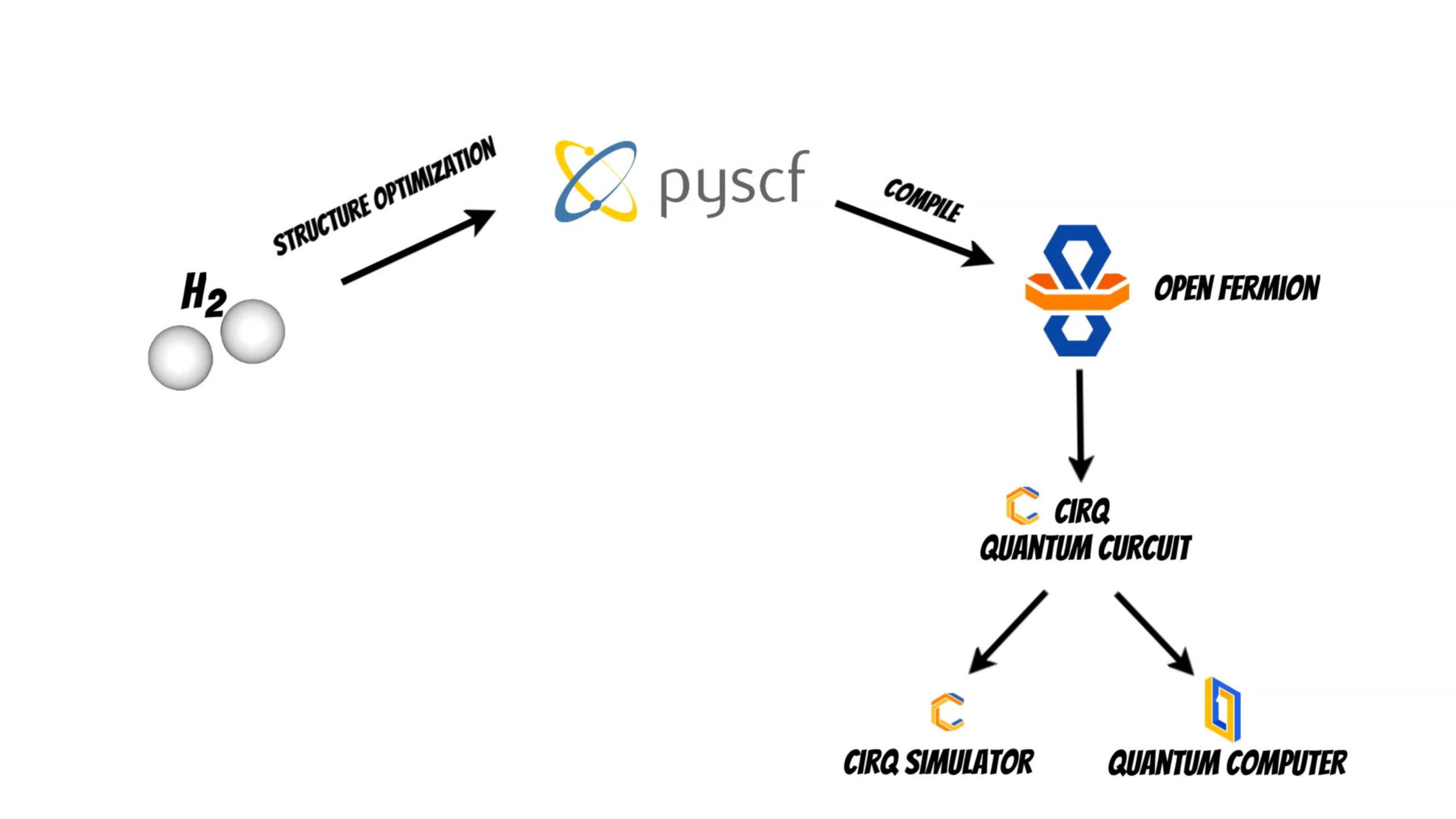# Bored with classical computers? – Quantum AI with OpenFermionIn this article I will show how we can prepare and perform calculations on quantum computers using OpenFermion, Cirq and PySCF.

Currently, there are many supercomputing centers, where we can run complicated simulations. However, there are still problems that are beyond the capabilities of classical computers, which can be addressed by quantum computers.Quantum chemistry and materials science problems which that are described by the laws of quantum mechanics can be mapped to the quantum computers and projected to qubits.

OpenFermion is the library which can help to perform such calculations on a quantum computer.

Additionally we will use the PySCF package which will help to perform initial structure optimization (if you are interested in PySCF package I have shared the example DFT based band structure calculation of the single layer graphene structure pyscf_graphene.ipynb).In our example we will investigate [latex]H_2[/latex] molecule for simplicity. We will use the PySCF package to find optimal bond length of the molecule.

Thanks to the OpenFermion-PySCF plugin we can smoothly use the molecule initial state obtained from PySCF package run in OpenFermion library (openfermionpyscf_h2.ipynb).

``````from openfermion.chem import MolecularData
from openfermionpyscf import run_pyscf

geometry = create_molecule(bond_length)
basis = 'sto-3g'
multiplicity = 1

run_scf = 1
run_mp2 = 1
run_cisd = 0
run_ccsd = 0
run_fci = 1

molecule = MolecularData(geometry, basis, multiplicity)

# Run pyscf.
molecule = run_pyscf(molecule,
run_scf=run_scf,
run_mp2=run_mp2,
run_cisd=run_cisd,
run_ccsd=run_ccsd,
run_fci=run_fci)

``````Now it is time to compile the molecule to the representation readable by the quantum computer using OpenFermion and Cirq library. Currently you can use several methods to achieve this:

Using one of this methods we get optimized quantum circuit. In our case the quantum cirquit for [latex]H_2[/latex] system will be represented by 4 qubits and operations that act on them (moment is collection of operations that act at the same abstract time slice).Finally we can use quantum circuit to run the calculations on the cirq simulator or on the real quantum computer.